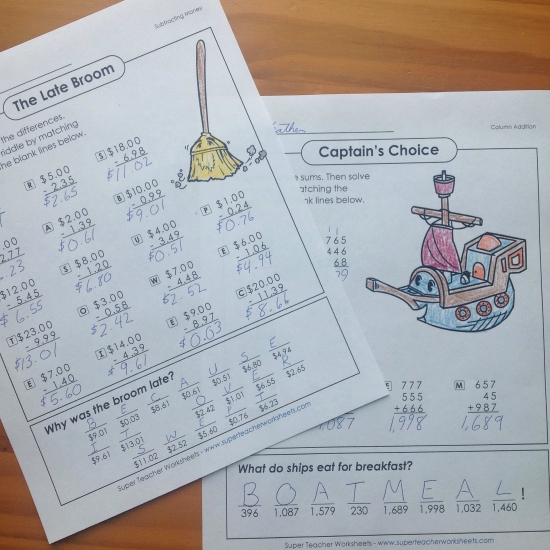# Math Riddles WorksheetsWe at Super Teacher Worksheets had some fun solving and coloring some of our new math riddles worksheets! We have a growing collection of math riddle printable worksheets for various math concepts. Students will love decoding the answer to a clever riddle by solving math problems. Our math riddle worksheets include practice for addition, subtraction, multiplication, division, Roman numerals, money, and place value. These are some great basic math skills worksheets, so be sure to check out our entire collection of math riddle worksheets!